Next: Multiple attenuation with the Up: Multiple attenuation: Theory and Previous: Signal and noise PEFs

# Multiple attenuation

When the noise and signal PEFs have been determined, the task of multiple attenuation follows. First consider that the seismic dataare the sum of signal (primaries) and noise (multiples):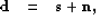(58)
whereis the signal we want to preserve andthe noise we wish to attenuate.

By definition of the signal and noise PEFs, the following relationships hold: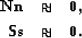(59)
Equations () and () can be combined to solve a constrained problem to separate signal from noise as follows:(60)
The scalaris related to the S/N ratio. In practice, however,is estimated by trial and error. Replacingbyin equation () yields(61)
Sometimes it is useful to add a masking operator for the noise and signal residuals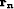and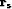when performing the noise attenuation. For example, in areas of the data where no multiples are present, the signal should be preserved. For instance, a mute zone can be taken into account very easily. Calling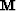this masking operator, the fitting goals in equation () are weighted as follows:(62)
Solving forin a least-squares sense leads to the objective function(63)
It is interesting to look at the least-squares inverse for: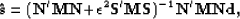(64)
where (') stands for the adjoint. Becauseis a diagonal matrix of zeros and ones,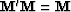. In practice, all computations are done in the time domain. In the Fourier domain, equation () demonstrates that the least-squares estimate ofis obtained by combining the spectra of both noise and signal. Abma (1995) shows that equation () is similar to Wiener filtering for random noise attenuation. Soubaras (1994) uses a very similar approach for random noise attenuation and more recently for coherent noise attenuation Soubaras (2001). Because the size of the data space can be quite large,is estimated iteratively with the conjugate-gradient method.

Therefore, multiple attenuation with prediction-error filters (in 2-D or 3-D) is two-step process where (1) noise and signal PEFs are estimated and (2) signal and noise are separated according to their multidimensional spectra. The next section illustrates this technique with the Sigsbee2B dataset.Next: Multiple attenuation with the Up: Multiple attenuation: Theory and Previous: Signal and noise PEFs
Stanford Exploration Project
5/5/2005